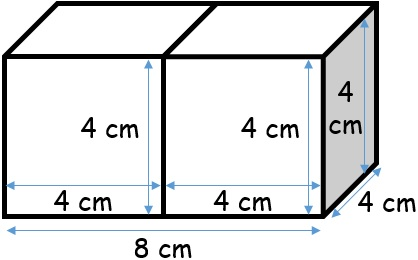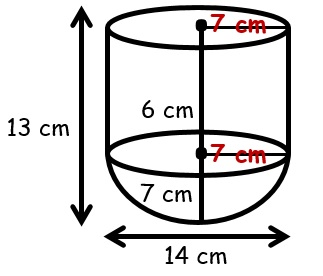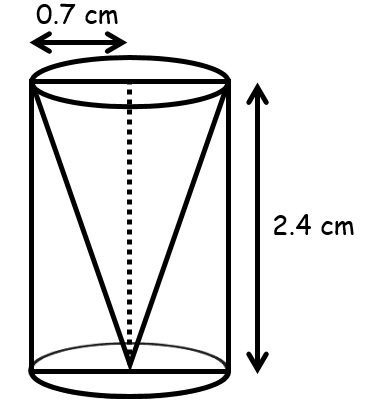# vs.eyeandcontacts.com

## Chapter 13 Surface Areas and Volumes Exercise 13.1

Question 1: 2 cubes each of volume 64 cm³ are joined end to end. Find the surface area of the resulting cuboid.Volume of cube = a³
= 64 cm³ = a³
= 64 = a
= 4 = a

Thus the length of each edge of cube is 4 cm. As both the cubes have same volume. Thus, both the cubes have same length, i.e., 4 cm.

Now as both the cubes are joined together, the length of cuboid = 8 cm, breadth = 4 cm, and height = 4 cm.

Therefore, the surface area of the cuboid so formed = 2(lb + bh + hl)
= 2[(8 x 4) + (4 x 4) + (4 x 8)]
= 2[32 + 16 + 32]
= 2
= 160 cm²

Thus, the surface area of the resulting cuboid is 160 cm².

Question 2: A vessel is in the form of a hollow hemisphere mounted by a hollow cylinder. The diameter of the hemisphere is 14 cm and the total height of the vessel is 13 cm. Find the inner surface area of the vessel.Radius of hemisphere, r = 7 cm (14/2 = 7 cm)
Height of cylinder, h = 13 - 7 = 6 cm

Therefore, the total inner surface area of the vessel = Inner surface area of hemisphere + Inner surface area of cylinder
= 2 π r² + 2 π r h
= 2 x (22/7) x 7² + 2 x (22/7) x 7 x 6
= 308 + 264
= 572 cm²

Thus, the inner surface area of the vessel is 572 cm².

Question 3: A toy is in the form of a cone of radius 3.5 cm mounted on a hemisphere of same radius. The total height of the toy is 15.5 cm. Find the total surface area of the toy.Height of the conical portion, h = 15.5 - 3.5 = 12 cm
Radius of the conical portion, r = 3.5 cm
Therefore, the slant height, l = √[r² + h²]
l = √[(3.5)² + (12)²]
l = √[12.25 + 144]
l = 12.5 cm

Therefore, the total surface area of the toy = Surface area of the conical portion + Surface area of the hemisphere
= [π x r x l] + [2 x π x r²]
= [(22/7) x 3.5 x 12.5] x [2 x (22/7) x 3.5 x 3.5]
= 137.5 + 77
= 214.5 cm²

Thus, the total surface area of the toy is 214.5 cm².

Question 4: A cubical block of side 7 cm is surmounted by a hemisphere. What is the greatest diameter the hemisphere can have? Find the surface area of the solid.As the hemisphere is surmounted on the cubical block, therefore, the hemisphere can have 7 cm as the greatest diameter.

Radius of the hemisphere, r = 3.5 cm (7/2 = 3.5 cm)

Therefore, the surface area of the solid formed = Curved surface area of cube + curved surface area of hemisphere - area of the base of hemisphere
= 6a² + 2 π r² - πr²
= [6 x (7)²] + [2 x (22/7) x (3.5)²] + [(22/7) x (3.5)²]
= 294 + 77 - 38.5
= 332.5 cm²

Thus, the surface area of the solid = 322.5 cm².

Question 5: A hemispherical depression is cut out from one face of a cubical wooden block such that the diameter l of the hemisphere is equal to the edge of the cube. Determine the surface area of the remaining solid.Edge of cube = l
Surface area of the cube = 6a²
= 6l²

Therefore, the radius of the hemisphere = l
Inner surface area of the hemisphere = 2 π r²
= 2 π (l/2)²
= 2πl²/4
= π l²/2

Area of circular portion of the hemisphere = π r²
= π (l/2)²
= π l²/4

Remaining surface area of the cubical box = Surface area of the cubical box + Inner surface area of the hemisphere - Area of circular region
= 6l² + π l²/2 - π l²/4
= 6l² + π l²/4
= l²/4 (24 + π)

Question 6: A medicine capsule is in the shape of a cylinder with two hemispheres stuck to each of its ends (see Fig. 13.10). The length of the entire capsule is 14 mm and the diameter of the capsule is 5 mm. Find its surface area.Radius of the cylindrical part of the capsule, r = 2.5 mm
Length of the cylinder, h = 9 mm

Total surface area of the capsule = Surface area of cylindrical part + 2(curved surface area of hemisphere)
= 2 π r h + 2(2 π r²)
= 2 π r (r + h)
= 2 x (22/7) x 2.5 [2 x 2.5 + 9]
= 5 x 22/7 x 14
= 220 mm²

Question 7: A tent is in the shape of a cylinder surmounted by a conical top. If the height and diameter of the cylindrical part are 2.1 m and 4 m respectively, and the slant height of the top is 2.8 m, find the area of the canvas used for making the tent. Also, find the cost of the canvas of the tent at the rate of ₹500 per m². (Note that the base of the tent will not be covered with canvas.)Radius of the cylindrical part, r = 2 m (4/2 = 2 m)
Height of the cylindrical part = 2.1 m

Curved surface area of the cylindrical part = 2 π r h
= 2 x (22/7) x 2 x 2.1
= 26. 4 m²

Curved surface area of the top = π r l
= 22/7 x 2 x 2.8
= 17.6 m²

Total area of the canvas = Curved surface area of cylindrical part + curved surface area of the conical part
= 26.4 + 17.6
= 44 m²

Cost of the canvas = ₹500/m²
Therefore, the total cost of the canvas = 44 x 500
= ₹22,000

Thus, the total cost of the canvas is ₹22,000.

Question 8: From a solid cylinder whose height is 2.4 cm and diameter 1.4 cm, a conical cavity of the same height and same diameter is hollowed out. Find the total surface area of the remaining solid to the nearest cm².Radius of the cylinder, r = 0.7cm (1.4/2 = 0.7 cm)
Height of the cylinder, h = 2.4 cm

Radius of cone, r = 0.7 cm
Height of cone, h = 2.4 cm
Slant height, l = ?
= l = √[(r)² + (h)²]
= l = √[(0.7)² + (2.4)²]
= l = √[0.49 + 5.76]
= l = 2.5 cm

Total surface area of the remaining solid = Outer curved surface area of cylinder + Area of bottom of cylinder + inner curved surface area of conical cavity
= 2π r h + pi r² + pi r l
= pi r (2h + r + l)
= (22/7) x 0.7 [2(2.4) + 0.7 + 2.5]
= 22 x 0.1 x [4.8 + 0.7 + 2.5]
= 17.6 cm² ≈ 18 cm²

Question 9: A wooden article was made by scooping out a hemisphere from each end of a solid cylinder, as shown in Fig. 13.11. If the height of the cylinder is 10 cm, and its base is of radius 3.5 cm, find the total surface area of the article.Given:
Height of the cylinder, h = 10 cm
Base radius of the cylinder, r = 3.5 cm

Therefore, the total surface area of the solid = curved surface area of cylindrical part + 2(inner surface area of hemispherical ends)
= 2pi r h + 2(2 pi r²)
= [2 x (22/7) x 3.5 x 10] + 2[2 x (22/7) x 3.5 x 3.5]
= 220 + 2(77)
= 220 + 154
= 374 cm²